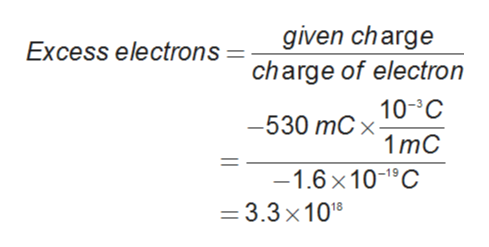# Calculate the number of protons and electrons for a charge of +610 mC and −530 mC respectively. Charge of electron is −1.6×10−19 C and charge of proton is 1.6×10−19 C. Hint: mC means milli coulomb. A)The excess number of protons is 3.8×1018.The excess number of electrons is 3.3×1018.B)The excess number of protons is 4.9×1018.The excess number of electrons is 1.3×1018.C)The excess number of protons is −3.1×1018.The excess number of electrons is −3.5×1018.D)The excess number of protons is 3.1×1018.The excess number of electrons is 3.5×1018.

Question
1 views

Calculate the number of protons and electrons for a charge of +610 mC and −530 mC respectively. Charge of electron is −1.6×10−19 C and charge of proton is 1.6×10−19 C. Hint: mC means milli coulomb.

A)The excess number of protons is 3.8×1018

.The excess number of electrons is 3.3×1018

.
B)The excess number of protons is 4.9×1018

.The excess number of electrons is 1.3×1018

.
C)The excess number of protons is −3.1×1018

.The excess number of electrons is −3.5×1018

.
D)The excess number of protons is 3.1×1018

.The excess number of electrons is 3.5×1018.

check_circle

Step 1

The electron and proton have electric charge.

The charge of the electron is -1.6×10-19C  and the charge of the proton is  .

The formula used for the calculation of excess protons and electrons is as follows:

Step 2

The number of protons is calculated as follows:

Step 3

The number of electrons is calculated as follows: ...help_outlineImage Transcriptionclosegiven charge charge of electron Excess electrons 10-C -530 mC x- 1 mC -1.6 x 10-1°C = 3.3 x1018 %| fullscreen

### Want to see the full answer?

See Solution

#### Want to see this answer and more?

Solutions are written by subject experts who are available 24/7. Questions are typically answered within 1 hour.*

See Solution
*Response times may vary by subject and question.
Tagged in

### Chemistry